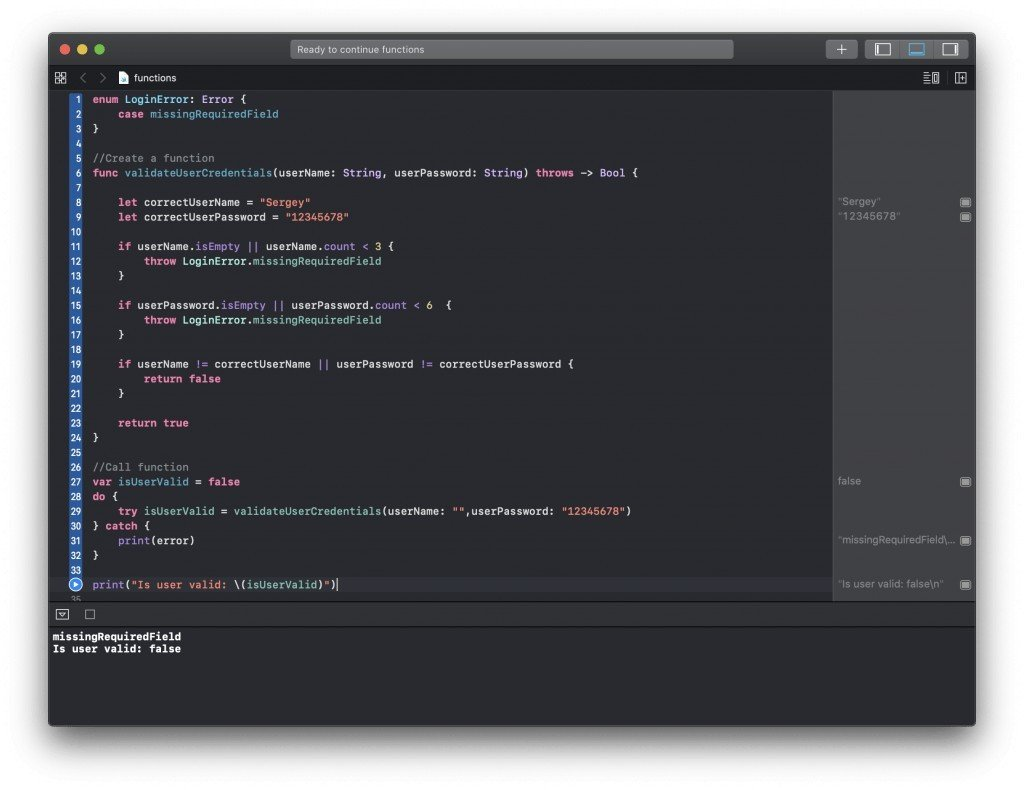# Swift Functions Tutorial with Code Examples

In this tutorial, you will learn how to work with functions in Swift.

## Create a New Function

The below code example demonstrates how to create a very basic function in Swift. We will be adding more details to it but in code examples below but for now, let’s learn how to create a very simple one. Functions in Swift start with a keyword func, then goes the function name, then go the parentheses and then the body of the function inside of curly brackets { }.

```func printHello() {
print("Hello")
}
```

To call the function I have created in the code snippet above, I will type the function name and add empty parentheses. Like so:

`printHello()`

I created the above function using Xcode Playground and here is how it looks when I run it.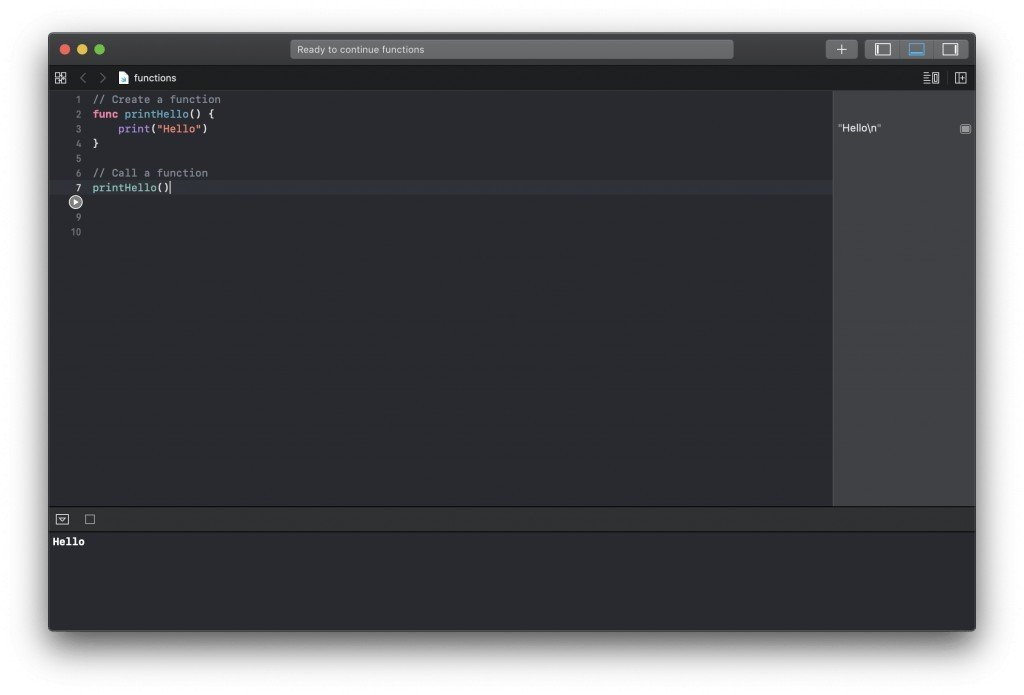## Function Parameters

Functions can accept parameters. In fact, functions can accept zero or as many parameters as you want to provide. Let’s first learn how to make a function that accepts two parameters.

Parameters are defined in function parentheses. To make a function accept a parameter, we will need to add to function parentheses a parameter name, and a parameter data type. Below is a code example of a function that accepts two parameters of String data type.

```func printFullName(firstName: String, lastName: String) {
print("\(firstName) \(lastName)")
}```

To call this function I will use:

`printFullName(firstName: "Sergey", lastName: "Kargopolov")`

Here is how this code looks in my Xcode Playground.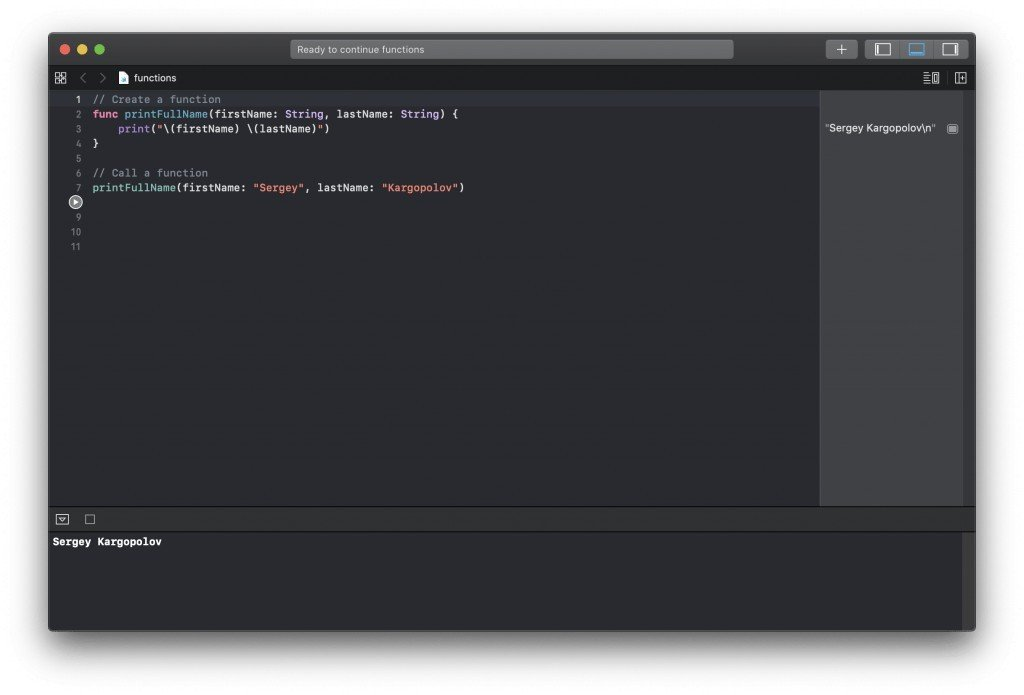## Parameter Label

Each parameter in a function can have a label. Parameter labels are used when a function is being called to make the function call more readable. Let’s add parameter labels to our printFullName function to see how they work.

```// Create a function
func printFullName(firstName firstNameValue: String, lastName lastNameValue: String) {
print("\(firstNameValue) \(lastNameValue)")
}

// Call a function
printFullName(firstName: "Sergey", lastName: "Kargopolov")
```

We declare parameter labels when we create a function, and we use parameter names when we make a function call. Parameter labels, make the function calls look more readable.

Here is how the code below looks and works in my Xcode Playground.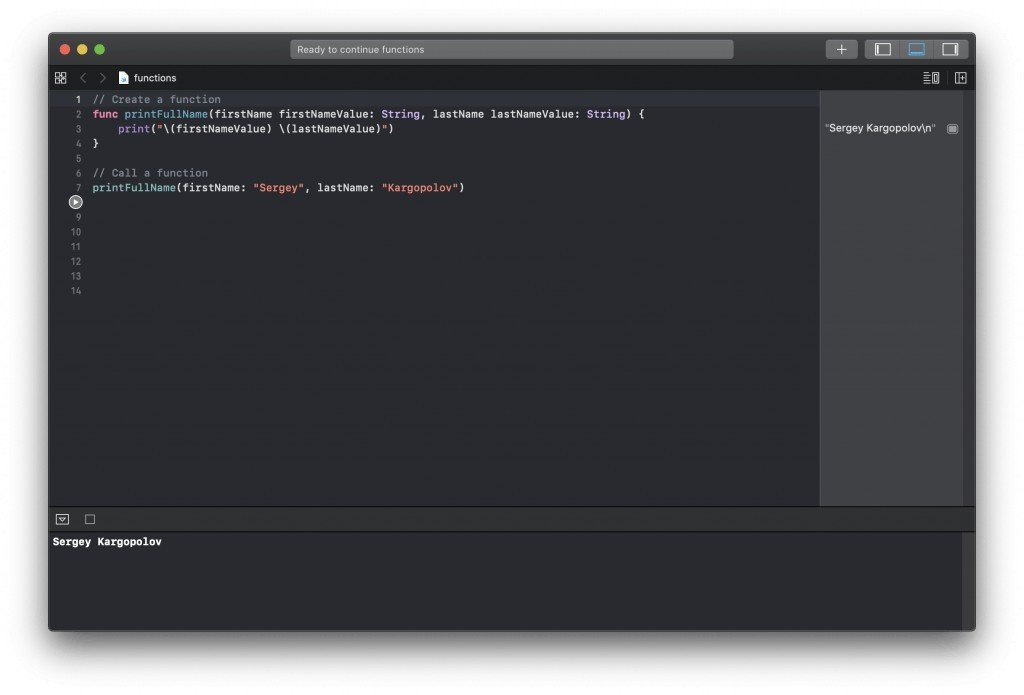## Omitting Parameter Labels

If needed parameter labels can be omitted. To omit parameter label we will need to use an underscore instead of a parameter label _ and then when calling a function to omit parameter label. Let’s have a look at the code example below:

```// Create a function
func getUserFullName(_ firstNameValue: String, _ lastNameValue: String) -> String {
return "\(firstNameValue) \(lastNameValue)"
}

// Call a function
let fullName = getUserFullName("Sergey", "Kargopolov")
print(fullName);```

Notice how the parameter labels have been replaced with underscores in the function name when it is created? And now because the parameter labels have been omitted, we do not use parameter labels in the function call as well. Below is a working example in Xcode Playground.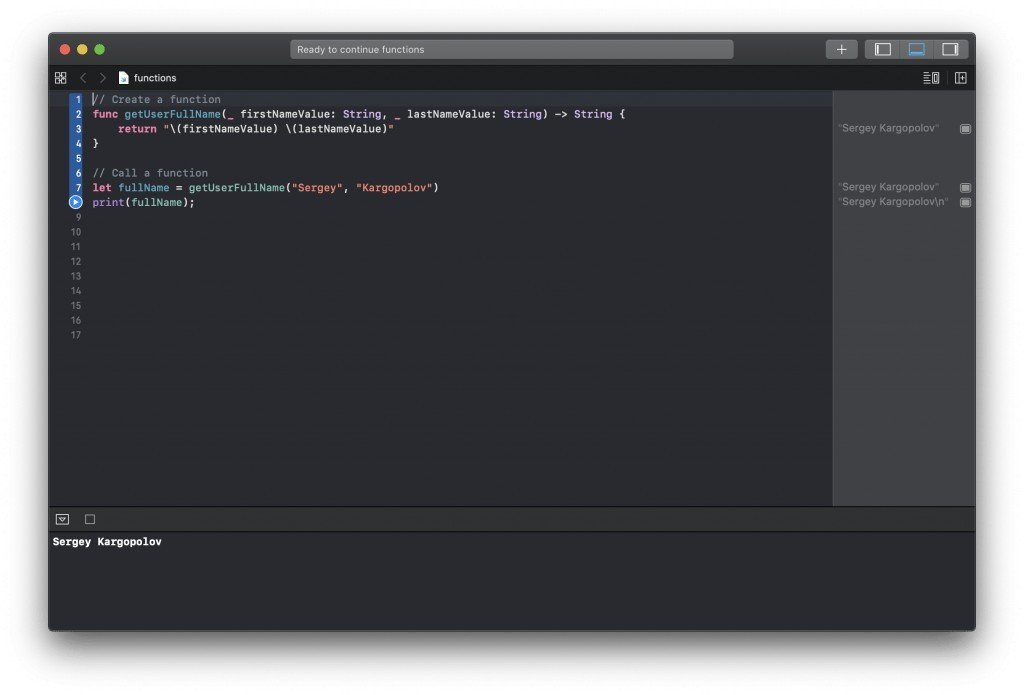## Default Parameter Value

Parameters can have a default value. A default parameter value is used when a value was not provided during the function call. We assign a default value to a parameter when we first define it in the function name. Let’s have a look at an example of a function that accepts two parameters and if parameter values were not provided, uses default values.

```func printTitles(page: Int = 1, limit: Int = 25) {
print("Printing titles for page: \(page)")
print("Max number of titles to print: \(limit)")
}```

In the above function printTitles an argument name page is assigned a default value of 1 which means that if this function does not receive value for this argument, a default value will be used.

Let’s call this function and not provide any of the parameters.

```// Parameters are not sent. Default values will be used.
printTitles()
```

As a result of this function call, default parameter values will be used.

```Printing titles for page: 1
Max number of titles to print: 25```

and now I will call this function and provide different parameter values:

```// Parameters are provided. Default values will not be used.
printTitles(page: 10, limit: 50)```

And the result will be:

```Printing titles for page: 10
Max number of titles to print: 50```

Below is a screenshot of my Xcode Playground demonstrating the work of the above function.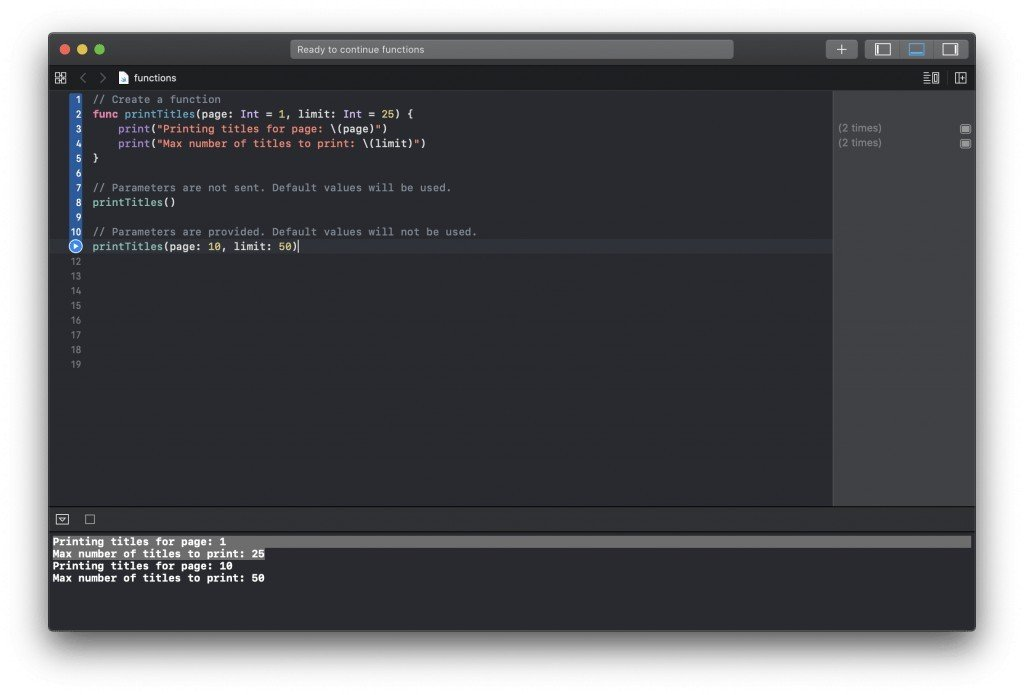## Returning a Value

A function can return a value. To return a value from a function we need to use the return keyword and also update the function name to specify the data type of the value a function can return. Let’s have a look at the example of a function that takes two parameters and returns a value.

```// Create a function
func getUserFullName(firstName firstNameValue: String, lastName lastNameValue: String) -> String {
return "\(firstNameValue) \(lastNameValue)"
}```

Notice how the function name has changed on the right side of it. To make a function return a value we needed to specify a data type of the value it is returning and the way we did it in the code example above is with -> String.

Here is how the above code looks in my Xcode Playground.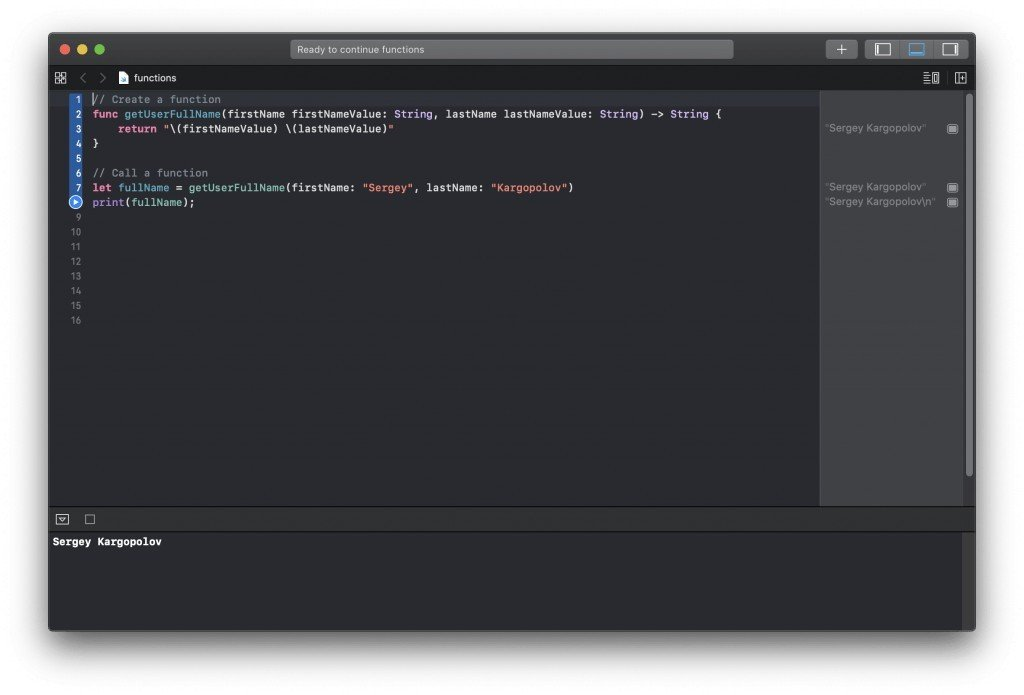## Functions That Throw Error

Swift functions can throw an error and you can write a code that catches that error and do something about it. To throw an error in Swift we use a throw keyword and to mark a function as throwing an error we use throws keyword in the function signature.

Below is an example of a Swift function that throws an error. Have a look at the function signature and see how the throws keyword is used. Also, go through the code of this function and notice how the throw keyword is used to throw an Error.

```//Create a function

}

}

return false
}

return true
}```

To call a function that throws an error we must use a do try and catch block. We put a try keyword on the line which calls a function that throws an error. As if we are saying “Try running this function“.

```//Call function
var isUserValid = false
do {